1. Introduction

For every investment, there exists an associated risk. In the world of investment markets, now a day, investors take a more conservative approach towards investments and take risks only when the expected return on the investment is known. This writing will shed light on the description of the Capital Asset Pricing Model, Capital Market Line, and Security Market Line. It will also highlight the importance of minimum variance portfolios and in the end, it will also discuss the significance of the CAPM equation for calculation of return.

1. Capital Asset Pricing Model (CAPM)

In the investment market, the relationship between systematic risk and expected return for the assets is of prime importance for investors. Capital Asset Pricing Model describes the relationship between two and shows that the return which an investor should expect from an investment is equal to the summation of risk-free return and risk premium (Fama and French 2004). Risk premium, in turn, is related to the beta coefficient of security. In the CAPM model, the beta coefficient of investment security is the measure of the volatility of returns or fluctuations of return. The graphical representation of CAPM is given as under;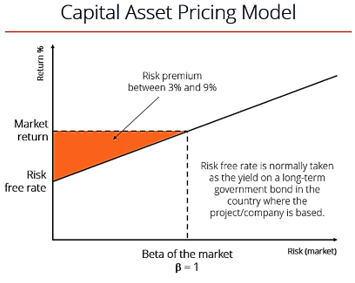1. Security Market Line (SML)

Security Market Line is a graphical representation of the Capital Asset Pricing Model (CAPM). The graph depicts the levels of risk against the expected return of the entire market at a given point in time (Frazzini and Pedersen 2014). The relationship between the expected return of a security and the associated measured risks are measured by “beta coefficient”. This means that when the beta security of a certain market portfolio will be zero then the expected rate of return will be equal to the risk-free rate. The equation of SML is stated as under;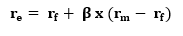Where re is the required return on an asset, rf is the risk-free rate, β is the beat coefficient. The graphical representation of SML is given below;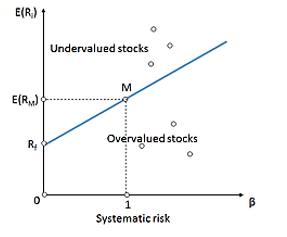1. Capital Market Line (CML)

Markowitz theory states that in case all the assets become risky then the optimal portfolio goes on to the efficient part of the frontier which in turn depends entirely on the attitude of the investor towards risk. As shown in the figure below, the market portfolio is along the concave frontier. The line intersecting the y-axis at point Rf, which is tangent to the concave set, is known as Capital Market Line (Bhojraj, Lee, and Oler 2003). Based on CML, it can be said that all the investors have the same opportunities and same tangency portfolio. So CML is the line which shows the expected rate of return of a market portfolio consisting of all proportions between market portfolio and a risk-free asset.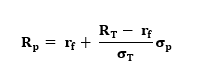Where p is a return on the portfolio, RT and σT are tangency portfolio T’s return and standard deviation. The factor ( ) is known as Sharpthe e Ratio. The graphical representation of CML is as follows;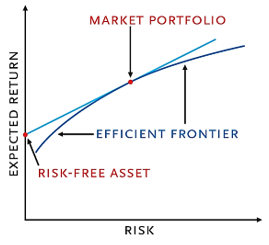1. Difference Between SML and CML

Security Market Line and Capital Market Line, both are important components of the Capital Asset Pricing Model. The main differences between these two lines are given as under;

• Risk & Rate of Return

Capital Market Line is basically the graphical representation of the CAPM model which shows the relationship between the risk-free rates of return and the levels of risk for a specific portfolio (Fama 1991). On the other hand, Security Market Line, also known as the characteristic line is the graphical representation of the relationship between the market’s risk and rate of return at a given time.

• Method of risk management

For the purpose of measuring risk associated with a certain portfolio at a given rate of return, Capital Market Line makes use of standard deviation or the total risk factor. Security Market Line, for the purpose of risk measurement, makes use of the beta coefficient to find the security’s risk contribution for the portfolio.

• Graphical Significance

As clear from the above graphical representation of Capital Market Line, it only defines the efficient portfolios. In other words, being superior to the efficient frontier, the CML only takes into account the inclusion of risk-free assets in the portfolio. On the other hand, the graphs of the Security Market Line define both efficient and non-efficient portfolios. The asset value is considered over-priced if its rate of return lies above the SML and vice versa.

• Portfolio and Stock

Capital Market Line determines the risks or returns rates for the efficient portfolios whereas Security Market line is used to determine the risk or rate of return for an individual stock.

• Systematic and non-systematic risks

Capital Market Line measures both systematic and unsystematic risks whereas Security Market Line only measures systematic risks only with the help of beta coefficient (Lakonishok and Shapiro 1986).

• Graphical Representation

As shown below, the Y-axis of the Capital Market Line represents the expected return and X-axis represents the standard deviation or the level of risk. On the other hand, the Y-axis of the Security Market Line represents the level of rate of return of particular assets and the X-axis shows the level of risk which in turn is measured by beta.

• Risk and Security Factors

In case of the measurement of risk factors, the Capital Market Line is considered superior to the Security Market linen but if the market portfolio and the risk-free assets are determined using Capital Market Line then all the security factors are measured or determined using Security Market Line.

1. Minimum Variance Portfolios

Such a portfolio of securities which can co bine to minimize the price volatility of the overall market portfolio is known as minimum variance portfolio. The terms “volatility” refers to the fluctuation of the security price in the investment community (Clarke, De Silva, and Thorley 2006). The ups and downs of the investment are directly related to the risks which it may encounter in the investment market. The basic need of developing minimum variance portfolios arise from the fact that the greater the volatility of investment market, the greater is its associated risks, based on that, an investor would want to develop minimum variance portfolio in order to minimize the ups and downs of the investment.

In Modern Portfolio Theory (MPT), the portfolio of risky assets at the starting point of the efficient frontier is the minimum variance portfolio, as shown in the graph below;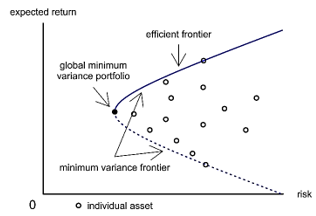1. Importance of Minimum Variance Portfolios

Majority of the minimum variance portfolios comprise of a mix of bonds and stock. Following points highlight the importance of minimum variance portfolios in the investment market;

• A diversified portfolio of securities

Portfolios are generally a set of investment securities which are held in one or different accounts by a certain investor. The concept of minimum variance portfolios compels the investor to have a combination of low volatility investments with are having low or no correlation with each other. The term “no correlation” refers to such investments which behave differently as compared to others when exposed to the same market and economic conditions. This forms the basis of the concept of minimum variance portfolios which in turn provide the investor with reduced volatility in investments.

• Reduced risks

Since minimum variance portfolio is located closer to the efficient frontier, as shown above in figure, it has a better sharp ratio i.e. risk-return ratio (Clarke, De Silva, and Thorley 2006). Diversification combined with the better risk-return ratio induces reduced volatility in the portfolio which in turn results in presence of a smaller number of risks to which the portfolio might be exposed in future. In this way, the minimum variance portfolio is known to significantly lower the losses in the course of market corrections.

• Higher return on portfolios

Market business studies show that the portfolio having lower price volatility or fluctuations are capable of achieving a better return. In the majority of the portfolios, these returns are even better than the average returns. The low volatility premium, which is an authentic tool for giving high returns on portfolios for years, can be optimally implemented using the minimum variance portfolios since the correlation between various investments, in that way, is also taken under consideration.

1. CAPM Equation for Rate of Return

By making use of the Capital Asset Pricing Model (CAPM), as discussed in the initial portion of the writing, the capital asset pricing model formula is given below. It can be divided into two parts i.e. the return rate free of risk and the risk premium of the specified security.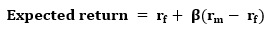Here the term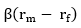is the indicative of risk premium. It is the difference between the market return and risk-free return, times the beta. Another formula for the Capital Asset Pricing Model (CAPM), is given below. This formula is utilized when regression analysis is applied to CAPM the model.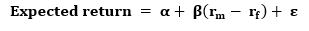Where α is the risk-free rate and ε is the error encountered while performing multiple regression.

1. CAPM Equation in Comparison with Alternate Equations

While calculating the rate of return, CAPM equation is most relevant than other asset pricing models based on the following key points;

• Assorted Portfolio

While calculating the rate of return, CAPM assesses both systematic and unsystematic risks associated with the investment. For an investor, diversification of the portfolio, in order to reduce the volatility of investment, is very important (Perold 2004). So, consideration of both systematic and unsystematic risks assists the investor in selecting the overall risk profile associated with the investment portfolio. This approach is more realistic and practical as compared to other asset pricing models since, in this way, the investor is able to safeguard the portfolio against potential risk factors which can reduce the overall profit over investment.

• Systematic Risk

With the help of the beta coefficient, the CAPM is able to take into account the systematic risk while depicting the relationship between total risk and expected return rate. This particular consideration is left out by most of the asset pricing models such as dividend discount model (DDM). Keeping in view the critical nature of unforeseen fluctuations in the investment market, systematic risk is of due importance while calculating the expected rate of return since the investor can prepare for mitigation measures in unfavorable scenarios (Estrada 2002).

• Business Risk vs Financial Risk

Another reason for relevancy of the CAPM equation for calculation of return rate is the fact that when business mix and the financing opportunities differ drastically then other asset pricing models cannot be used to carry out return related calculations such as weighted average cost of capital (WACC), which in turn can easily affect the return prospect of investment. By using CAPM, investors can calculate the exact risk factor in order to assess the differential valuations.

1. Conclusion

Capital Asset Pricing Model shows the relationship between systematic risk and the expected return for the assets. It can be graphically represented with the help of the Security Market Line which in turn shows the same i.e. the levels of risks against the expected returns of the entire market at a given point. Security Market Line shows such a relationship with the help of beta coefficient which is the measure of the volatility of the investment portfolio.

The Capital Market line is such a representation of CAPM which shows the relationship between risk-free rates of return and levels of risk for a specified portfolio whereas Security Market Line shows the relationship between same at a given instant. CML uses standard deviation for measuring investment associated risks whereas SML makes use of beta-coefficient. CML only defines efficient portfolios whereas SML defines both efficient and inefficient portfolios.

Such a portfolio which combines to minimize the price volatility is known as minimum variance portfolio. Minimum Variance Portfolio provides a diversified portfolio of securities which in turn results in reduced risks and higher returns for investors. The CAPM equation is the most relevant equation while calculating returns since it makes use of assorted portfolio and considers systematic risks which are of critical importance for investors.

1. References

Bhojraj, Sanjeev, Charles MC Lee, and Derek K Oler. 2003. “What’s my line? A comparison of industry classification schemes for capital market research.”  Journal of Accounting Research 41 (5):745-774.

Clarke, Roger, Harindra De Silva, and Steven Thorley. 2006. “Minimum-variance portfolios in the US equity market.”  Journal of Portfolio Management 33 (1):10.

Estrada, Javier. 2002. “Systematic risk in emerging markets: the D-CAPM.”  Emerging Markets Review 3 (4):365-379.

Fama, Eugene F. 1991. “Efficient capital markets: II.”  The journal of finance 46 (5):1575-1617.

Fama, Eugene F, and Kenneth R French. 2004. “The capital asset pricing model: Theory and evidence.”  Journal of economic perspectives 18 (3):25-46.

Frazzini, Andrea, and Lasse Heje Pedersen. 2014. “Betting against beta.”  Journal of Financial Economics 111 (1):1-25.

Lakonishok, Josef, and Alan C Shapiro. 1986. “Systematic risk, total risk and size as determinants of stock market returns.”  Journal of Banking & Finance 10 (1):115-132.

Perold, André F. 2004. “The capital asset pricing model.”  Journal of economic perspectives 18 (3):3-24.

## ESSAY Instant Price

Get an Instant Price. No Signup Required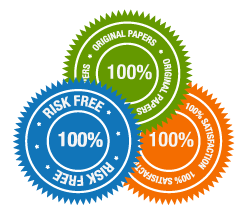### Contact Us At### Subscribe

Join our mailing list today and benefit from our free ebooks, daily deals, and discount